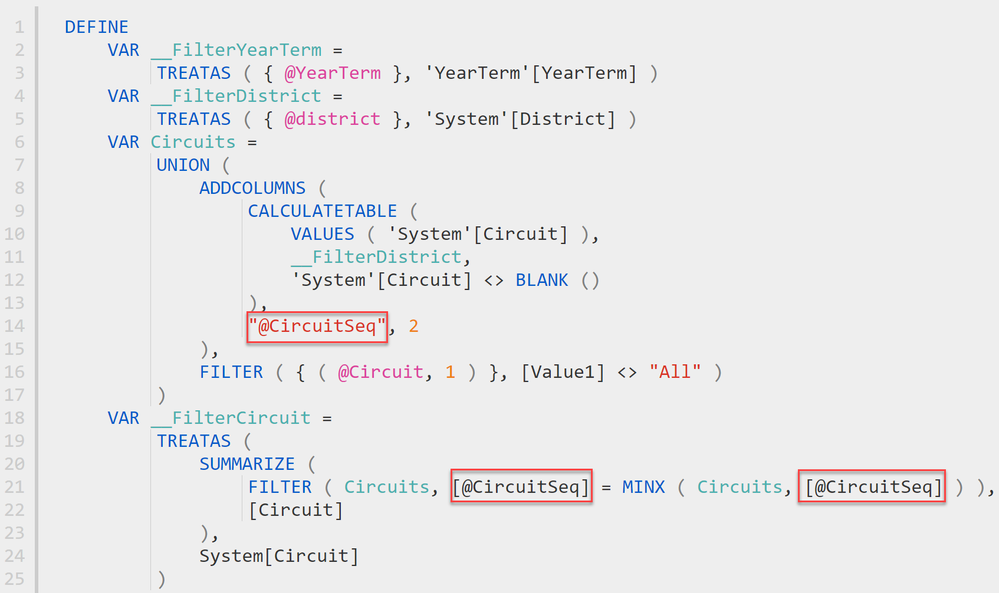cancel
Showing results for
Did you mean:Regular Visitor

## Sytnax Error Rabbit Hole

Hello all,

I am developing a report in Power BI Report Builder and I am running into a syntax error that formats just fine on daxformatter, so it must be something else. The issue is with VAR Clusters. Please see below for the query. Interesting enough, if you remove VAR Clusters and VAR __FilterCluster, the query compiles just fine. I have been copy pasting the Circuit section and replacing it with my Cluster stuff. Circuits and Clusters are defined to have the same inputs and the query's are practically identical ( a little different in the Hierarchy order). Any help is greatly appreciated because I am losing my mind at this point.

I renamed "Clusters" to "Cluxter" and it works. Real curious as to why Clusters is not accepted?

DEFINE
VAR __FilterYearTerm =
TREATAS ( { @YearTerm }, 'YearTerm'[YearTerm] )
VAR __FilterDistrict =
TREATAS ( { @district }, 'System'[District] )
VAR Circuits =
UNION (
CALCULATETABLE (
VALUES ( 'System'[Circuit] ),
__FilterDistrict,
'System'[Circuit] <> BLANK ()
),
"Circuits[Seq]", 2
),
FILTER ( { ( @Circuit, 1 ) }, [Value1] <> "All" )
)
VAR __FilterCircuit =
TREATAS (
SUMMARIZE (
FILTER ( Circuits, Circuits[Seq] = MINX ( Circuits, Circuits[Seq] ) ),
[Circuit]
),
System[Circuit]
)
VAR Clusters =
UNION (
CALCULATETABLE (
VALUES ( 'System'[Cluster] ),
__FilterCircuit,
'System'[Cluster] <> BLANK ()
),
"Clusters[Seq]", 2
),
FILTER ( { ( @Cluster, 1 ) }, [Value1] <> "All" )
)
VAR __FilterCluster =
TREATAS (
SUMMARIZE (
FILTER ( Clusters, Clusters[Seq] = MINX ( Clusters, Clusters[Seq] ) ),
[Cluster]
),
System[Cluster]
)
VAR Schools =
UNION (
CALCULATETABLE (
VALUES ( 'System'[SystemKey] ),
__FilterCluster,
'System'[SystemKey] <> BLANK ()
),
"Schools[Seq]", 2
),
FILTER ( { ( @School, 1 ) }, [Value1] <> -99 )
)
VAR __FilterSchool =
TREATAS (
SUMMARIZE (
FILTER ( Schools, Schools[Seq] = MINX ( Schools, Schools[Seq] ) ),
[SystemKey]
),
System[SystemKey]
)
VAR Phases =
UNION (
FILTER (
SUMMARIZECOLUMNS ( Grade[Phase], "Phases[Seq]", 2 ),
),
FILTER ( { ( @Phase, 1 ) }, [Value1] <> "All" )
)
VAR __FilterPhase =
TREATAS (
SUMMARIZE (
FILTER ( Phases, Phases[Seq] = MINX ( Phases, Phases[Seq] ) ),
[Phase]
),
)
UNION (
CALCULATETABLE (
__FilterPhase,
),
),
FILTER ( { ( @Grade, 1 ) }, [Value1] <> -99 )
)
TREATAS (
SUMMARIZE (
),
)
VAR __DS0Core =
SUMMARIZECOLUMNS (
'YearTerm'[YearTerm],
'System'[SystemKey],
'System'[Province],
'System'[Region],
'System'[District],
'System'[Circuit],
'System'[Cluster],
'System'[SchoolName],
'LearnerChart'[LearnerKey],
'LearnerChart'[Surname],
'LearnerChart'[FirstName],
'LearnerChart'[TermPromotion],
'Subject'[Description],
__FilterCircuit,
__FilterCluster,
__FilterSchool,
__FilterYearTerm,
"ReportMarks", [ReportMarks],
"DaysAbsent", CALCULATE ( AVERAGE ( 'LearnerChart'[DaysAbsent] ) ),
"AverageReportMark", CALCULATE ( AVERAGE ( 'LearnerChart'[Mark] ) ),
"ReportMarkLevel", CALCULATE ( AVERAGE ( 'LearnerChart'[RmLevel] ) ),
"AverageFailures", CALCULATE ( AVERAGE ( 'LearnerChart'[Failures] ) ),
"AverageNearFailures", CALCULATE ( AVERAGE ( 'LearnerChart'[NearFailuresLC] ) ),
"AverageDistinctions", CALCULATE ( AVERAGE ( 'LearnerChart'[Distinctions] ) ),
)

EVALUATE
__DS0Core

The Parameter Cluster is defined to be a text input. Here is the query for the Cluster parameter.

EVALUATE
UNION (
SUMMARIZECOLUMNS (
System[Circuit],
System[Cluster]
),
{ ( "!All", "All" ) }
)
ORDER BY System[Cluster]

Query for Circuit Parameter

EVALUATE
UNION (
SUMMARIZECOLUMNS (
System[District],
System[Circuit]
),
{ ( "!All", "All" ) }
)
ORDER BY System[Circuit]

4 REPLIES 4Community Champion

@MacBI You are trying to access columns from a Variable which isn't supported.

Circuits[Seq] = MINX ( CircuitsCircuits[Seq] )

The Circuits[Seq] isn't a valid syntax.Regular Visitor

Hmm, I'm not sure I agree with you. In my variable Circuits I have this defined.

VAR Circuits =
UNION (
CALCULATETABLE (
VALUES ( 'System'[Circuit] ),
__FilterDistrict,
'System'[Circuit] <> BLANK ()
),
"Circuits[Seq]", 2
),
FILTER ( { ( @Circuit, 1 ) }, [Value1] <> "All" )
)

As mentioned above, I renamed my variable "Clusters" to "Cluxter" and everything compiled. My paginated reports are now working as intended.Community Champion

@MacBI You're right. I used DAX Formatter it assumed that those were variable refrerences so I relied on it, didn't notice you used the Table[Column] notation in the new column name.The best practice while naming columns is to use "@" so that readers can easily distinguish between Model Column, Virtual Column and a Measure.Regular Visitor

Got it. I will start doing that going forward, thanks!